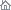Home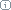About Us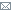ContactBlogWhat's NewProductsBuy NowDownloadsForumGeneXproTools Online Guide Learn how to use the 5 modeling platforms of GeneXproTools with the Online Guide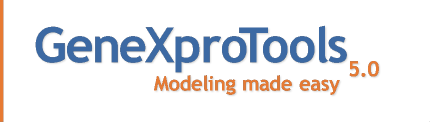Last update: February 19, 2014Quantile Regression

Quantile Tables are by themselves powerful analytics tools, but they are also at the heart of the Logistic Regression Model and Logistic Fit. In addition, they are also the basis of powerful analytics tools such as Gains and Lift Charts, which are essential for making good decisions about the quality of a model and to estimate the benefits of using a model.

The number of quantiles or bins is entered in the Quantiles combobox at the top of the Logistic Regression Window. The most commonly used Quantile Tables such as Quartiles, Quintiles, Deciles, Vingtiles, Percentiles, and 1000-tiles are listed by default, but you can type any valid quantile number in the box to build the most appropriate quantile table for your data.

The number of quantiles is an essential parameter for most of the analyses performed in the Logistic Regression Window (obviously Quantile Regression and Analysis, but also Gains Chart, Lift Chart, Log Odds and Logistic Regression, Logistic Fit, and Logistic Confusion Matrix) and therefore it is saved for each model (the number of bins is in fact an essential parameter of all Logistic Regression fitness functions and therefore it can also be changed in the Fitness Functions Tab of the Settings Panel).

On their own, Quantile Tables are widely used in risk assessment applications and in a variety of response models to create rankings or scores. Percentiles, for instance, are very popular and often used for that purpose alone. But in GeneXproTools, Quantile Tables are also used to create a more sophisticated ranking system: the probabilistic ranking system of the Logistic Regression Model. This model estimates unique probabilities for each case, forming a very powerful ranking system, perfectly bounded between 0 and 1.

GeneXproTools shows its Quantile Tables in 100% stacked column charts, where the distribution of both Positive and Negative categories is shown for all the bins. By moving the cursor over each column, GeneXproTools shows both the percentage and absolute values for each class. For more than 20 bins, a scroll bar appears at the bottom of the Quantile Chart and by moving it you can see the distribution over all the range of model outputs.

Besides allowing the visualization of Quantile Tables, GeneXproTools also shows and performs a weighted Quantile Regression. Both the slope and intercept of the regression line, as well as the R-square, are computed and shown in the Quantile Regression Chart.

These parameters form the core of the Quantile Regression Model and can be used both to evaluate rankings and to make discrete classifications in a fashion similar to what is done with the Logistic Regression Model. Within the Logistic Regression Framework of GeneXproTools, however, only the Logistic Regression Model is used to evaluate rankings (probabilities, in this case) and to estimate the most likely class. Furthermore, the Scoring Engine of GeneXproTools also uses the Logistic Regression Model to make predictions, not the Quantile Regression Model.

Note also that in the X-axis of the Quantile Regression Chart, GeneXproTools plots model outputs and therefore you can see clearly how spread out model scores are. Note also that, in the Quantile Regression Chart, upper boundaries are used if the predominant class is “1” and the model is normal, or the predominant class is “0” and the model is inverted; and lower boundaries are used if the predominant class is “1” and the model is inverted, or the predominant class is “0” and the model is normal.

On the companion Statistics Report shown on the right in the Logistic Regression Window (the Quantiles section opens up every time the Quantiles Chart Tab is selected), GeneXproTools also shows the Spread from Top to Bottom, Spread from Top to Middle, and Spread from Middle to Bottom (when the number of bins is even, the middle value is the average of the two middle bins). Note that negative values for the spreads, especially the Spread from Top to Bottom, are usually indicative of an inverted model. In absolute terms, however, the wider the spread the better the model.

Related Tutorials:

Related Videos:

 Leave Feedback Please enter the number below using the combo boxes before sending your feedback. 3 8 4 0 1 2 3 4 5 6 7 8 9   0 1 2 3 4 5 6 7 8 9   0 1 2 3 4 5 6 7 8 9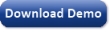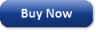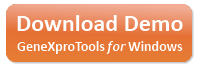Time Limited Trial

Released February 19, 2014

Last update: 5.0.3883New Entries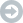Subscribe to the GEP-List

 3 8 4 0 1 2 3 4 5 6 7 8 9   0 1 2 3 4 5 6 7 8 9   0 1 2 3 4 5 6 7 8 9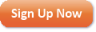Home | What's New | Products | Buy Now | Downloads | Quick Tour | Support | Contact Us | About Gepsoft | Sign UpForum | Blog | Videos | Tutorials | Server Knowledge Base | Logistic Regression Guide | Terms of Use | Privacy & Cookies